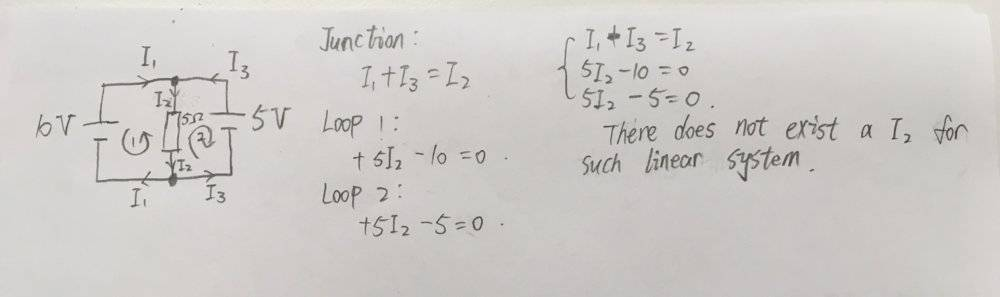# Kirchhoff's rule for circuit with two batteries

Homework Statement:
I found a difficulty solving the following question using Kirchhoff's rules.
Relevant Equations:
Kirchhoff's rules.SammyS
Staff Emeritus
Homework Helper
Gold Member
Problem Statement: I found a difficulty solving the following question using Kirchhoff's rules.
Relevant Equations: Kirchhoff's rules.

View attachment 242850
Correct. The solution is: There is no solution.

To confirm this"
Taking the "outer" loop, in a clockwise direction (The loop containing only the 10V and 5V sources.) gives you:

10V − 5V = 0.

Correct. The solution is: There is no solution.

To confirm this"
Taking the "outer" loop, in a clockwise direction (The loop containing only the 10V and 5V sources.) gives you:

10V − 5V = 0.

That's the thing that bothers me a lot. What is the physical reality that corresponds to no solution? does that mean no current anywhere or what?

SammyS
Staff Emeritus
Homework Helper
Gold Member
That's the thing that bothers me a lot. What is the physical reality that corresponds to no solution? does that mean no current anywhere or what?
No. It's a physically impossible situation if those are "ideal" sources of voltage. ##I_1 \to \infty## and ##I_3 \to -\infty## .

It's much like a short circuit.

•cnh1995 and QuantumRose
No. It's a physically impossible situation if those are "ideal" sources of voltage. ##I_1 \to \infty## and ##I_3 \to -\infty## .

It's much like a short circuit.
Thank you!

cnh1995
Homework Helper
Gold Member
This is one of the invalid connections in circuit theory. You can't connect two unequal ideal voltage sources in parallel and two unequal ideal current sources in series.

•berkeman and QuantumRose
This is one of the invalid connections in circuit theory. You can't connect two unequal ideal voltage sources in parallel and two unequal ideal current sources in series.
This clears my mind, thank you

•berkeman